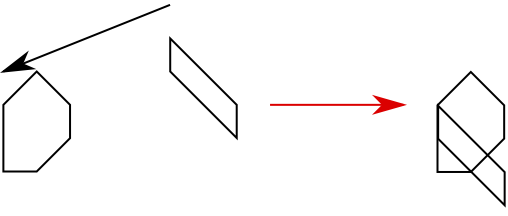시간 제한 메모리 제한 제출 정답 맞은 사람 정답 비율
5 초 256 MB 0 0 0 0.000%

## 문제

Yandex company, as well as many other companies around the world, allows students to get an experience of working as a developer in a large software company by offering internship options to them. All the interns are being interviewed (almost) like real programmers, they are being asked for their knowledge of algorithms, for their understanding of OOP principles and for many other things mostly depending on the area the internship candidate wants to work on.

You are dreaming about working in the team of Yandex.Maps. As a team that is closely related to geography, the developers of Maps face lots of geometric problems that involve dealing with complex regions on the world maps. One of the questions that they may ask\footnote{Actually not, relax :)} you during an interview is described in the next paragraph.

Consider two convex polygons located on the plane. You are allowed to shift the second polygon arbitrarily without any rotations or reflections. Write a program that finds a shift vector for the second polygon such that the area of the union of the first polygon and the shifted second polygon equals to exactly $S$, or determine that it is not possible.

This position is all you need, so don't lose your chance and solve this problem before they think you are incapable of such easy exercises!

## 입력

The first line contains two integers $n$ and $m$ and a real value $S$ ($3 \leq n, m \leq 2000$, $0 \leq S \leq 10^{13}$), the number of vertices in each of the polygons and the desired union area. The area is given with at most ten digits after the decimal point.

Each of the next $n$ lines contains two integers $x_{1,i}$ and $y_{1,i}$ ($-10^6 \leq x_i, y_i \leq 10^6$): the coordinates of the vertices of the first polygon in counter-clockwise order.

The next $m$ lines describe the second polygon in a similar format.

It is guaranteed that both polygons are strictly convex: no three vertices of any polygon lie on the same line.

It is also guaranteed that for any $S'$ such that $\frac{|S' - S|}{\max\{1, |S|\}} \leq 10^{-3}$, the desired shift vector exists or not exists exactly as for the given $S$.

## 출력

On the first line, output either "Yes" or "No" depending on if it is possible to find such a shift vector that the area of union of polygons equals to $S$.

If the desired shift vector exists, on the second line, output two real numbers $\mathit{dx}$ and $\mathit{dy}$ that denote the coordinates of a shift vector of a second polygon.

Your answer will be considered correct if the area of union of the first polygon and the second polygon shifted by your vector has absolute or relative error of no more than $10^{-4}$ with respect to $S$. That is, if $\hat{S}$ is the exact area value that is calculated by your shift vector, the answer will be considered correct if $\frac{|\hat{S} - S|}{\max\{S, 1\}} \leq 10^{-4}$.

## 예제 입력 1

6 4 5.25
0 0
1 0
2 1
2 2
1 3
0 2
5 4
5 3
7 1
7 2


## 예제 출력 1

Yes
-5.0 -2.0


## 예제 입력 2

4 4 2.5
0 0
2 0
2 1
0 1
0 5
1 5
1 7
0 7


## 예제 출력 2

No


## 힌트

The illustration for the first sample test is given below: